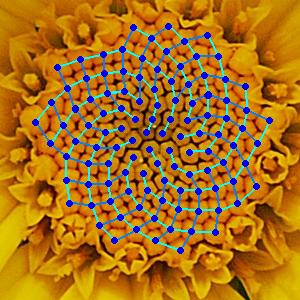# Is math related to science

## Applied mathematics in the natural sciencesThe development of mathematics is closely linked to that of the natural sciences. For example, the development of analysis under Leibniz and Newton would be unthinkable without the simultaneous advances in the natural sciences, such as classical mechanics. Until well into the 20th century there was no clear separation between mathematicians and natural scientists: Newton, Leibniz or Georg Simon Ohm were "universal scholars" who studied mathematics and physics as well as philosophy. In all natural sciences one encounters mathematical phenomena that are not all are obvious at first glance. In botany, for example, the famous Fibonacci numbers are suitable for describing the spiral arrangement of leaves around a stem axis in order to optimize the incidence of light. And central terms in algebra and geometry form the basis of combinatorial chemistry. Using mathematical models, new structural formulas for chemical products in medicine and technology are generated and analyzed. The closest relationship exists between mathematics and physics, since mathematics is, as it were, the "language into which physics translates the laws of nature". Due to the increasingly available computing power of modern computers, the simulation of physical processes is gaining in importance in addition to purely theoretical research. This means that the job market is increasingly looking for generalists who are not only able, like all mathematicians, to think in a structured and conceptual way, but who are also prepared for the constantly evolving IT world.

### Applied mathematics in the natural sciences at Bavarian universities

The focus of these courses is the mathematical modeling and simulation of scientific and technical processes.